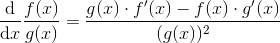# AP Calculus AB : Understanding the derivative of a sum, product, or quotient

## Example Questions

### Example Question #1 : Understanding The Derivative Of A Sum, Product, Or Quotient

Find the derivative of the following function: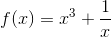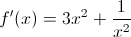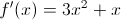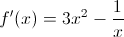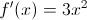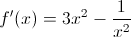Explanation:

Since this function is a polynomial, we take the derivative of each term separately.

From the power rule, the derivative of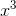is simply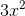We can rewrite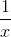as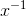and using the power rule again, we get a derivative of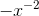or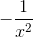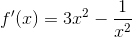### Example Question #2 : Understanding The Derivative Of A Sum, Product, Or Quotient

What is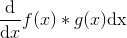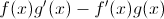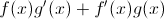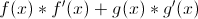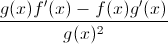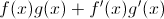Explanation:

The chain rule is "first times the derivative of the second plus second times derivative of the first".

In this case, that means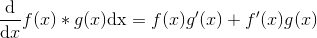.

### Example Question #3 : Understanding The Derivative Of A Sum, Product, Or Quotient

Which of the following best represents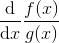?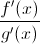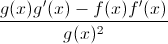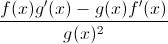Explanation:

The question is just asking for the Quotient Rule formula.

Recall the Quotient Rule is the bottom function times the derivative of the top minus the top function times the derivative of the bottom all divided by the bottom function squared.

Given,the bottom function is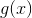and the top function is. This makes the bottom derivative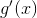and the top derivative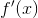.

Substituting these into the Quotient Rule formula resulting in the following.